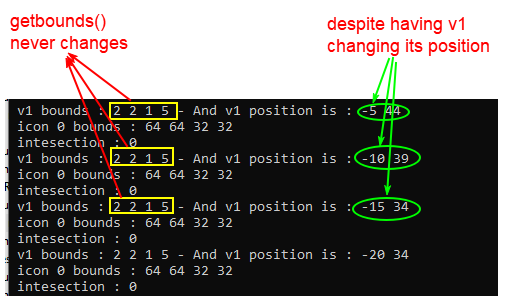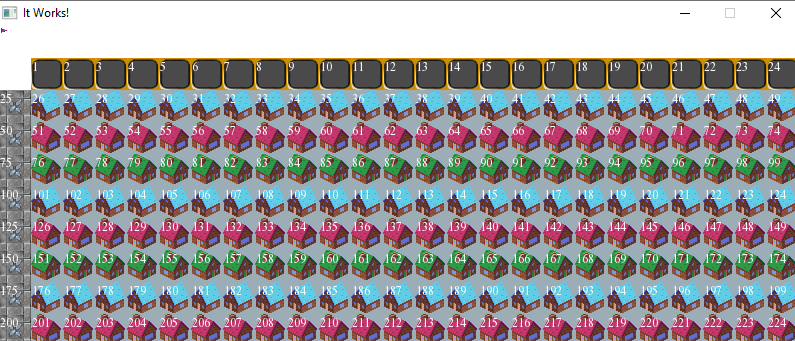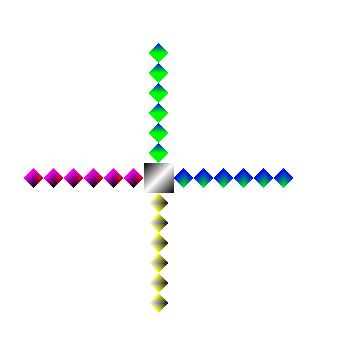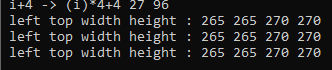###Author Topic: Inhereting from Transformable+Drawable+VertexArray: getBounds/draw error  (Read 5300 times)

0 Members and 1 Guest are viewing this topic.

#### Power##### Inhereting from Transformable+Drawable+VertexArray: getBounds/draw error
« on: April 24, 2020, 05:51:34 pm »
Hello,
I don't know if this should be in the "window help" section or here, but the problem i am presensting here, started when i wanted to get the Bounding boxes of my entities.

I made a class using vertices and wanted to get the bounding boxes through the function "getBounds" by inhereting the class from the "VertexArray" class, like this :
class VertexClass :  public sf::VertexArray, public sf::Transformable , public sf::Drawable
{
private: /// ///////////////////////////////////////////
sf::VertexArray V;
sf::Texture Tex;
virtual void draw(sf::RenderTarget& target, sf::RenderStates states) const    {
states.transform *= getTransform();
states.texture = &Tex;
//states.texture = nullptr;
target.draw(V, states);
}
public:/// ////////////////////////////////////////////////////////////////////////////////////////////////
bool VertexFunction(sf::Vector2f v0,sf::Vector2f v1,sf::Vector2f v2 ) //(...,PrimitiveType type)
{
V.setPrimitiveType(sf::Triangles);
V.resize(3);

V.position = v0;
V.position = v1;
V.position = v2;
V.texCoords = v0;
V.texCoords = v1;
V.texCoords = v2;
return true;
}
};

One problem when calling the "draw" function :
//main
VertexClass VClass;
VClass.VertexFunction(sf::Vector2f(200,200),sf::Vector2f(400,200),sf::Vector2f(400,400));

....
window.draw(VClass);

Error message is :
Quote
'sf::Drawable' is an ambiguous base of 'VertexClass'

I searched on the internet and found these solutions : https://www.ibm.com/support/knowledgecenter/en/SSLTBW_2.1.0/com.ibm.zos.v2r1.cbclx01/cplr138.htm

Maybe i did not get it but i tried the following options :
window.sf::Drawable::draw(VClass);
//or
window.sf::VertexClass::draw(VClass);
I tried then to modify the class declaration, and added "sf::Drawable" before the draw function declaration. Still have the problem..

1) So how do we solve this situation if anyone knows?
2) Is there a better method to get the bound box of my verticesClass? (This is way i choose to put the post in the graphics section of the forum).

« Last Edit: April 26, 2020, 12:44:38 pm by Power »

#### Laurent

• Hero Member
••• Posts: 32504##### Re: Inhereting from Transformable+Drawable+VertexArray: getBounds() and draw() error
« Reply #1 on: April 24, 2020, 06:38:49 pm »
Public inheritance is not a solution here -- as you noticed, it brings more problems than solutions.

What's the problem with this?
class VertexClass
{
sf::FloatRect getBounds() const {return m_geometry.getBounds();}

sf::VertexArray m_geometry;
}
Laurent Gomila - SFML developer

#### Power##### Re: Inhereting from Transformable+Drawable+VertexArray: getBounds() and draw() error
« Reply #2 on: April 24, 2020, 06:53:32 pm »
Public inheritance is not a solution here -- as you noticed, it brings more problems than solutions.

What's the problem with this?
class VertexClass
{
sf::FloatRect getBounds() const {return m_geometry.getBounds();}

sf::VertexArray m_geometry;
}

Perfect! Worked perfecetly fine. SFML is fun, but i don't know when to use "const" yet, i shall use sf variable more often.
Thank you

#### Power##### GetBounds Gives always the same results
« Reply #3 on: April 26, 2020, 01:20:17 pm »
Hello, i met with a problem : "GetBounds Gives always the same results"

My first SFML tutorial was the making of Pong game. It used the "getGlobalBounds" from the Shape class, and "intersects" from the Rect class. The ball AND the pad from the PONG game Were moving .. So the result of the intrescetion was sometimes "1" sometimes "0".

Fast forward to the example of this forum post, i have a VertexArray and i am using the "getBounds()" function. The problem simplly IS : "When The vertex shape MOVES, its bounds "getbounds values" are not changing. "

I thought maybe it was because the Getbound function was a "const", i redeclared it inside the vertexClass as a function without "const", and it did not change the result : getbounds gives the same results whichever is the position of the vertex shape.

Let's take an example, here is the class and its 2 functions (the  : "Contruction4noTex()" and "getBounds()" :
class VertexFun :   public sf::Transformable , public sf::Drawable
{
private: /// ///////////////////////////////////////////
sf::VertexArray V;
sf::Texture Tex;
virtual void draw(sf::RenderTarget& target, sf::RenderStates states) const    {
states.transform *= getTransform();
states.texture = &Tex;
//states.texture = nullptr;
target.draw(V, states);
}///
public:
bool Contruction4noTex(sf::Vector2f v0,sf::Vector2f v1,sf::Vector2f v2,sf::Vector2f v3,sf::Color c0, sf::Color c1,sf::Color c2,sf::Color c3)
{

V.resize(4);

return true;
}
sf::FloatRect getBounds() {return V.getBounds();}
};

Declaration of a vertex shape called "v1" inside the main :
VertexFun v1;
v1.Contruction4noTex(sf::Vector2f(2,2),sf::Vector2f(3,2),sf::Vector2f(3,7),sf::Vector2f(2,7),red,blue,red,magenta);
v1.move(sf::Vector2f(0,64)); // giving the shape its initial position

Testing the intersection of the shape called v1 and a FloatRect :

/// Testing bounds

sf::FloatRect Rect3(64,64,32,32);
float a1= v1.getBounds().left;
float a2= v1.getBounds().top;
float a3= v1.getBounds().width;
float a4= v1.getBounds().height;
float b1= Rect3.left;
float b2= Rect3.top;
float b3= Rect3.width;
float b4= Rect3.height;

cout << " v1 bounds : " << a1 << " "  << a2 << " "  <<a3 << " "  <<a4 << " - And v1 position is : " << v1.getPosition().x << " " << v1.getPosition().y << endl;
cout << " icon 0 bounds : " << b1 << " "  << b2 << " "  <<b3 << " "  <<b4 << endl;
bool c1 = v1.getBounds().intersects(Rect3);
cout << " intesection : " << c1 << endl;

As you can see i i tried to follow the values on the console using "std::cout" while watching the position of the shape while it is moving.

Here is what the console shows :The intersection result is "0" but if i defined Rect3 as (0,0,32,32) the result would be "1" but that's not the problem, the problem is getbounds() gives always the same values despite v1 moving, what i am missing?

#### Laurent

• Hero Member
••• Posts: 32504##### Re: Inhereting from Transformable+Drawable+VertexArray: getBounds/draw error
« Reply #4 on: April 26, 2020, 02:55:11 pm »
The position is changed with sf::Transformable::setPosition. The bounds are given by sf::VertexArray::getBounds(). How would these two things interact with each other without you doing something? How would you get a changing bounding rect magically without doing anything in your class?

sf::VertexArray::getBounds() simply returns the min/max of the vertices' coordinates. If you never change them, then the bounding rect will never change as well.

On the other hand, changing the position changes the translation components inside sf::Transformable, and in result, modifies the entity's transform (getTransform()).

Now... after correctly understanding all that stuff, how to write a getGlobalBounds() function that works similarly to SFML entities' getGlobalBounds()? Simply by transforming the vertex array bounds by the entity's transform. And taking the bounding rect of the result.

I really think you're going too fast. You're trying complicated stuff (it is!) without having a solid background (you thought it was caused by the "const" keyword, which doesn't make any sense).

Can't you work with SFML entities directly, until you understand SFML better? Why do you create your own entity class? What are you trying to achieve, what's your final goal?
Laurent Gomila - SFML developer

#### Power##### Re: Inhereting from Transformable+Drawable+VertexArray: getBounds/draw error
« Reply #5 on: April 26, 2020, 05:01:46 pm »
The position is changed with sf::Transformable::setPosition. The bounds are given by sf::VertexArray::getBounds(). How would these two things interact with each other without you doing something? How would you get a changing bounding rect magically without doing anything in your class?

sf::VertexArray::getBounds() simply returns the min/max of the vertices' coordinates. If you never change them, then the bounding rect will never change as well.

On the other hand, changing the position changes the translation components inside sf::Transformable, and in result, modifies the entity's transform (getTransform()).

Now... after correctly understanding all that stuff, how to write a getGlobalBounds() function that works similarly to SFML entities' getGlobalBounds()? Simply by transforming the vertex array bounds by the entity's transform. And taking the bounding rect of the result.
Very interesting! After reading your answer i tried "doing something" to changes the 4 variables defining the FloatRect obtained through getBounds(), so i did the following :

1) Store the FloatRect obtained with getbounds in 4 variables.
2) Each time the vertex moves, modify the first and second variables which were the initial "left" and "top" components of the floatRect. I never modify the "width" or "height" variables.
3) Use a FloatRect that contains the new "left" and "top" variables with the old width and height variables, and use it directly with "intesects".

So whenever my object moves, i get a FloatRect similar to the getGlobalBounds() i think? It seems to be working.

___ Now let's move to phase 2
Now to understand SFML better as you are suggesing me, maybe i should try to do it with "getTransform()) as you suggest.
To sum up, the transformable class (which my class derives from ) has the ability to use getTransform(), it generates an object from the class sf::Transform.
Its functions https://www.sfml-dev.org/documentation/2.5.1/classsf_1_1Transform.php
Seems to have very few "getters" (but probably good enough), i don't see a getter that would give me information that can be applied to the vertexArrayObject.getBounds() to change it... I am sure there is but i don't see it.
It's probably inside the "getMatrix () const" function? which gives a matrix of four variables. I am supposed to use these 4 variables and apply them to the FloatRect obtained with GetBound(), then use the resulting FloatRect, i think?

Happax showed me a link to understand the 3x3 matrix and it's effects, i guess i have to dig more, but yeah i used another method and left the getTransform methode you are telling me about unfortunately, i would like to be able to do the method you are describing.

I really think you're going too fast. You're trying complicated stuff (it is!) without having a solid background (you thought it was caused by the "const" keyword, which doesn't make any sense).

Can't you work with SFML entities directly, until you understand SFML better? Why do you create your own entity class? What are you trying to achieve, what's your final goal?
True, since i understood how to inherit from the Transformable class i felt i can do anything.. and tried to do anything. Yes i am moving too fast, that's becuase i feel i am being too slow.

So my goal (for now) is as follows :
--- I have a big map containg.. let's say.. houses, like this :---Each house has a FloatRect.
---My vertixClass allows me to create any shape, the little object in the upper left is the shape (it's not exactly a triangle, it's a combinason of three VertixArray's).
I am using a vertexClass because i want it be able to expand the entity and make it smaller at will, and i want it to have a specific shape, convex and concave. That's why i am not using the regular "shape" entities that SFML offers such as RectangularShape etc..

---What i want to do?
When my entity moves and reach a certain floatRect (which can be the green house or the red one or anything), something happens : a sound plays or the house is destroyed.

To test if the entity reached the house, you have to use the "intersects" function, and you have to obtain the entity bounds with getBounds (hence the beginning of this forum post).

So you can figure out the rest, i had a problem because getBounds did not give me a different FloatRect for each entity position, your solution  seems to be interesting which is to play with getTransform() and which i am not sure how to use, in the meantime i tried to "save" all the "movements" the entity does, if the item goes "right" i can predict if it has reached a floatRect.. something like that. And it did the trick somehow. Not sure if it's the best way to do it?
Thank you for your answers and time, by the way. It's great to have this forum.
« Last Edit: April 26, 2020, 05:09:10 pm by Power »

#### Laurent

• Hero Member
••• Posts: 32504##### Re: Inhereting from Transformable+Drawable+VertexArray: getBounds/draw error
« Reply #6 on: April 26, 2020, 06:11:53 pm »
I forgot to say: don't hesitate to have a look at how other SFML entities implement similar stuff.

Look at sf::Sprite:

FloatRect Sprite::getGlobalBounds() const
{
return getTransform().transformRect(getLocalBounds());
}
Where getLocalBounds() is equivalent to vertexArray.getBounds() in your case.

sf::Transform is a transformation, its final goal is to transform things. So that's how you're supposed to use it, and I think the API documentation is more than enough to find out what you can transform with it -- in your case, a sf::FloatRect. The 'S' in SFML is for 'Simple', so don't go in crazy directions, like wondering how to use the transform's matrix elements directly...Quote
To test if the entity reached the house, you have to use the "intersects" function
That's just a convenience function to quickly check if two aligned rectangles intersect. As you can guess, it won't give accurate results for testing a triangle (or any other polygonal shape). But then, it depends what your requirements are.
Laurent Gomila - SFML developer

#### Power##### Re: Inhereting from Transformable+Drawable+VertexArray: getBounds/draw error
« Reply #7 on: May 02, 2020, 06:36:34 pm »
..
I had a very very hard time trying to try to use the sf::sprite getglobal bounds idea. Also i moved to another method, and removed "sf::sprite" from the function you suggested and tried what follows (it was supposed to be posted in a different post but i got an error while posting)

Hello, I am getting an error message trying to post a new post on the forum and it says : report it to the admin if it happens again, which did.
Here the content of the post so i dont lose it :
Quote
Hello, so here is the shape i made, and my goal ultimately is to be able to test the intersections between some entity and the elements of this image specifically (it means : not the rectangle surrounding the shape).Its construction follow the tilemap example, its has a vertexArray as an attributes which is resized to match the size of the total numbers of vertices needed to make the shape in the image above. So total numbers of vertices is :
1) +4 (for the rectangle in the middle)
2) +(6*4) for the diamond shapes on every side.
3) multiply line 2 by 4.
Totat vertices = 4+(6*4)*4= 100.

I proceed to construct the vertices with a function member of the class
class VertexFun :  public sf::Transformable , public sf::Drawable
{private:

sf::VertexArray V;
...}
And the function would be :
public :
{...} /// i dont need the first parameter, it's just in case i want to use a texture

Final result is shown at the beginning of this post.
VertexFun shape1;
....
window.clear(sf::Color::White);

window.draw(shape1);
window.display();

Okay now i am taking the advice i was given lastly here : https://en.sfml-dev.org/forums/index.php?topic=27161.0
And i am trying to get the global bounds of the vertexArray:

i implemented this function member :
sf::FloatRect getGlobalBounds()
{
return getTransform().transformRect(V.getBounds());
}

And when using it in the main :
sf::FloatRect ff;
ff=shape1.getGlobalBounds();
int aa,bb,cc,dd;
aa=ff.left;bb=ff.top;cc=ff.width;dd=ff.height;
cout << " left top width height : " << aa << " " << bb << " " << cc << " " << dd << " " << endl;

I find that it has what i presume the bound of the rectangle made by the whole shape :AND, when i use a moving function:
up = (sf::Keyboard::isKeyPressed(sf::Keyboard::Up) ? 1 : 0);
if(up==1)
{
shape1.move(0,-10);
cout << " left top width height : " << aa << " " << bb << " " << cc << " " << dd << " " << endl;
}
The bounds are the same.
___
To the questions :

1) I think i should have a member function (sf::FloatRect getGlobalBounds()) that applies to each little structures (the rectangle + diamonds) i made, and then proceed to test them ALL, to be able to check the "intersection" of an entity with my vertexclass?
But IS THAT POSSIBLE? Since i have only one "VertexArray" in my Class? The GetBounds applies to the wholes points of the vertexArray and not individually for each 4 vertices.... (reminder : all the elements in the image are 4-vertices shapes inside ONE vertexAray)

2) Any other idea how to do it?
Thanks
« Last Edit: May 02, 2020, 06:42:17 pm by Power »

#### Laurent

• Hero Member
••• Posts: 32504##### Re: Inhereting from Transformable+Drawable+VertexArray: getBounds/draw error
« Reply #8 on: May 02, 2020, 07:04:28 pm »
If I sum up... you have all these quads in a single entity, but you'd like to test intersection with each of them rather than with the whole bounds of the entity?

So yes, obviously you should test each quad. This is possible, you have the coordinates of the 4 vertices of each quad, as well as the transform. From that you can either get an AABB for each quad, using code similar to what we said previously, or transform each vertex and perform a more precise test against a custom concave shape (using the separate axis theorem -- Google it).
Laurent Gomila - SFML developer

#### Power##### Re: Inhereting from Transformable+Drawable+VertexArray: getBounds/draw error
« Reply #9 on: May 02, 2020, 08:00:28 pm »
If I sum up... you have all these quads in a single entity, but you'd like to test intersection with each of them rather than with the whole bounds of the entity?
Yes exactly Mister Laurent.

So yes, obviously you should test each quad. This is possible, you have the coordinates of the 4 vertices of each quad, as well as the transform. From that you can either get an AABB for each quad, using code similar to what we said previously, or transform each vertex and perform a more precise test against a custom concave shape (using the separate axis theorem -- Google it).

I found it : https://gamedevelopment.tutsplus.com/tutorials/collision-detection-using-the-separating-axis-theorem--gamedev-169 OOh myy this seems to be like quite a read that i can probably complete, with time.

1) Let's say i made the same shape but with regular sf::RectangularShape 's. And i used "rotate" to make the "diamonds" in the side.

Will getGlobalBounds() for the rotated RectangularShapes (the diamonds) give me different results when it has been rotated and do i have to use a function similar to the one you explained (using getTransform and TransformRect) etc.. ? In any case i need the theorem you quoted to do the test boundaries right?

2)
Using rectangularShapes to make lines and make a shape like the one in this image (the entity in the upper right)
If i made it, will i be able to check the bounds of an item passing through easily? I am suspecting that getlobalBounds() will give me a very big rectangle and not the a zone describing precisely the lines i have drawn.

#### Power##### Re: Inhereting from Transformable+Drawable+VertexArray: getBounds/draw error
« Reply #10 on: May 02, 2020, 08:02:29 pm »
I forgot to add the image for the entity of the question number 2:#### Laurent

• Hero Member
••• Posts: 32504##### Re: Inhereting from Transformable+Drawable+VertexArray: getBounds/draw error
« Reply #11 on: May 03, 2020, 08:14:38 am »
It seems like you are mixing two very different things: rendering and physics. Don't try to rely only on SFML functions to perform your intersection tests; bounding rects are there for convenience (quick/preliminary tests), you can't build you whole physics on them.

Therefore, don't choose how to draw your entities according to that. Figure out what kind of intersection tests you need (rectangle/line, circle/concave, whatever...), Google it, and don't be afraid to write some code, it's not that complicated and you'll learn very interesting and useful stuffLaurent Gomila - SFML developer

#### Power##### Re: Inhereting from Transformable+Drawable+VertexArray: getBounds/draw error
« Reply #12 on: May 06, 2020, 12:46:11 am »
Okay thank you for the answer.
Feedback : I feel there should be a whole tutorial about the physics and all that you described.

Or at least 2 sentences in the "Bounding boxes" section of the "Position, rotation, scale: Transforming entities" tutorial, mentioning the necessity of studing specific interactions outside of the tutorials when it comes to non-rectangular entities. Because at first i imaging being able to test collisions of any kind of entity prociving i could use SFML functions such as getGlobalBounds() etc.. someone might have the same assumption.
« Last Edit: May 06, 2020, 12:48:33 am by Power »

anything